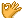•闪存
•博客
• 发言小组
• 投递新闻
• 提问博问
• 添加收藏
•文库

3

##20哪位大神帮忙看一下，小白不是很懂这里，x = 5是局部变量还是全局变量？

def a(): x = 5 def b(): x = 4 print(x) return b() a() 4 def a(): x = 5 def b(): x = x + 5 print(x) r